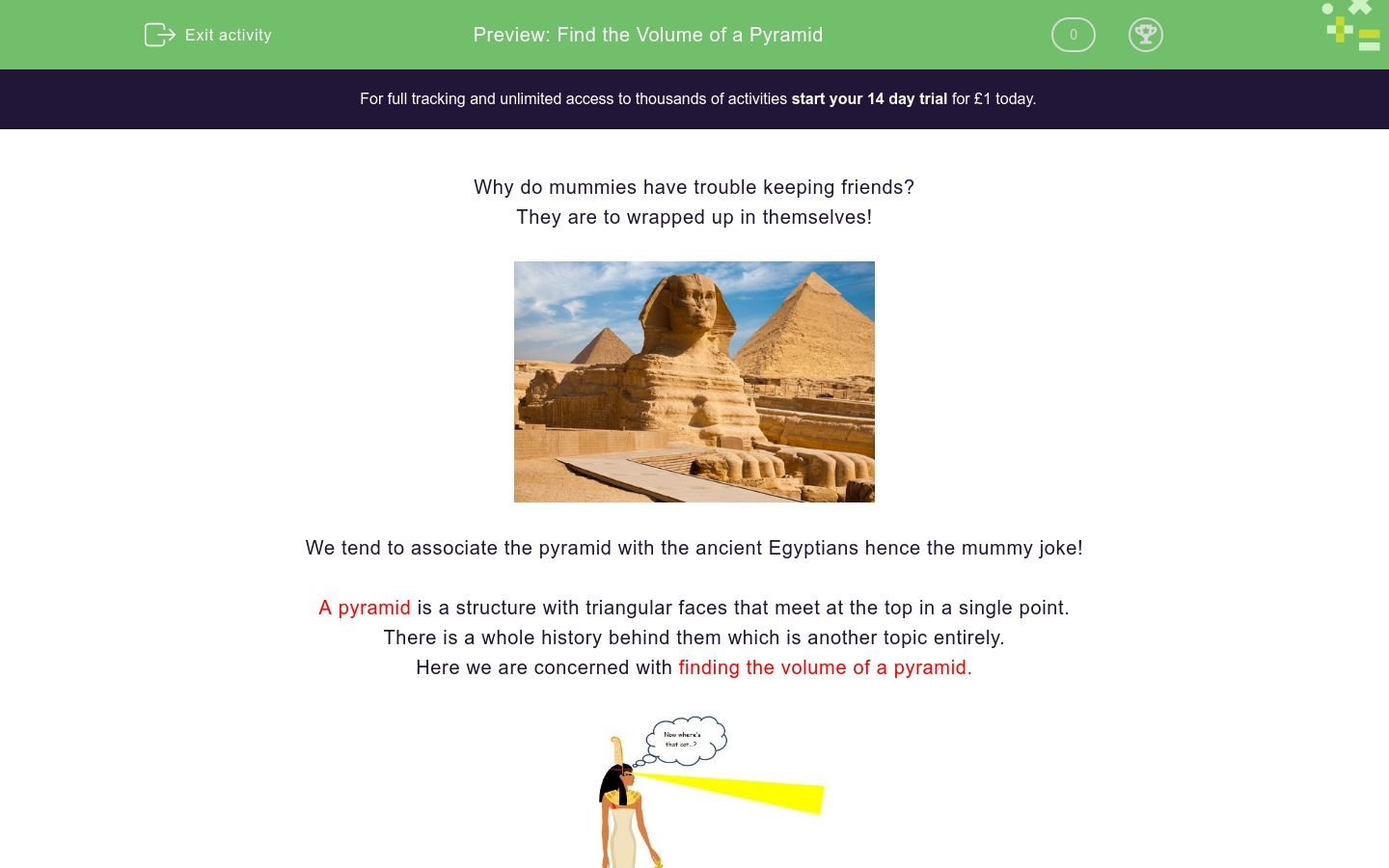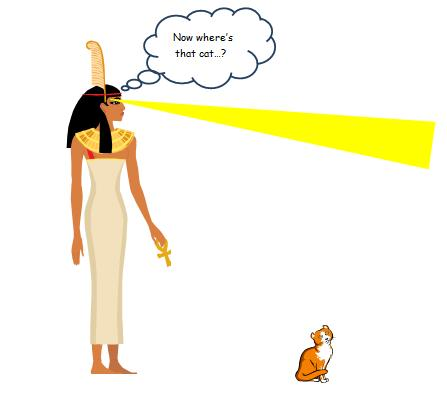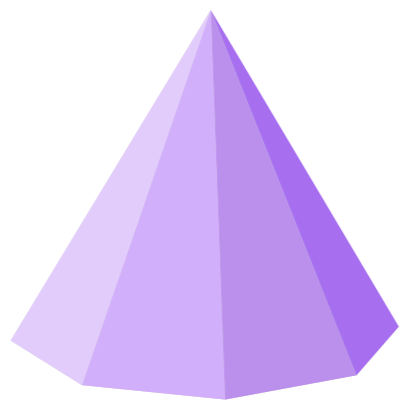# Find the Volume of a Pyramid

In this worksheet, students will learn the formula for finding the volume of a pyramid and be able to apply it.Key stage:  KS 4

GCSE Subjects:   Maths

GCSE Boards:   AQA, Eduqas, Pearson Edexcel, OCR

Curriculum topic:   Geometry and Measures, Mensuration

Curriculum subtopic:   Mensuration and Calculation, Volume and Surface Area Calculations

Difficulty level:### QUESTION 1 of 10

Why do mummies have trouble keeping friends?

They are to wrapped up in themselves.

I can think of worse ways to introduce the topic of pyramids, believe me I can.We tend to associate the pyramid with the ancient Egyptians hence the mummie joke.

A pyramid is a structure with triangular faces that meet at the top in a single point.

There is a whole history behind them which is another topic entirely.

Here we are concerned with finding the volume of a pyramid.Ancient Egyptians were clever people ..generally.... and left us a formula to work with to find the volume.

Volume of a pyramid = 1/3 x base area x height.

The base area could be any shape. You will need to know how to find the area of basic shapes such as square, rectangle, and triangle.

To enter our examples you need to solve thisHow do you use an ancient Egyptian doorbell?

Toot and come inFind the volume of this pyramid.  The rectangle has sides of 3 cm and 4 cm. The height is 6 cm.

Find the area of the base first.

3 x 4 = 12 cm²

Now put everything into you calculator at once.

1 ÷ 3 x 12 x 6 = 24 cm³

We use units³ because we are working with volume.

I think we have this all wrapped up now.What is the volume of this square based pyramid of sides 4 cm and height 3 cm?What is the volume of this rectangular based pyramid of sides 4 cm and 6 cm and height 5 cm?These Christmas tree baubles have been made up into a pyramid.

What is the volume?

42.48 units³

58.27 units³

41.66 units³

39.50 units³What is the area of this rectangular based pyramid?

Sides are 9m and 8 m and the height is 6 m.

138 m³

134 m³

148 m³

144 m³

What is the formula for finding the volume of a pyramid?

1/3 x base x Height &divid; 2

1/3 x base area x Height

1/3& divide; base area x Height

1/3 x base area x Height ÷3;An octagonal based pyramid has a base area of 24 cm² and is 12 cm tall.

1/3 x base x Height &divid; 2

1/3 x base area x Height

1/3& divide; base area x Height

1/3 x base area x Height ÷3;

The base of this pyramid measures 35 m by 35 m.  It is 20.6 m high.What is the volume of this​ pyramid rounded to the nearest whole number?

There are lots of interesting facts and figures surrounding pyramids. You can actually go inside them. The volume that we are interested in is 8416 m³, 8415 m³ or 8412 m³These three square based pyramids all have the same base area but vary in height.

Base = 15 m

Height of pyramid 1 is 6 m

Height of pyramid 2 is 7 m

Height of pyramid 3 is 11 m

Find the following

 450 m³ 540 m³ 555 m³ 525 m³ 825 m³ 852 m³ 375 m³ Volume of 1 Volume of 2 Volume of 3 Difference in volume between 1 and 3square based pyramid has sides of 9 cm and height 9 cm?

Another one is going to be attached underneath to make an ornamental garden hanging.

What is the volume of the ornament?This square based pyramid has a volume of 94.2 cm³

The base area is 51.84 cm²

What is the height of the pyramid?

• Question 1What is the volume of this square based pyramid of sides 4 cm and height 3 cm?

16
EDDIE SAYS
Did you remember to calculate the area of the base first. As it is square based we know all sides are the same. Area = 4 x 4 = 16 Now put it into your formula. 1 ÷ 3 x 16 x 3 = 16
• Question 2What is the volume of this rectangular based pyramid of sides 4 cm and 6 cm and height 5 cm?

40
EDDIE SAYS
Did you remember to calculate the area of the base first. As it is rectangular based multiply the length of both sides first. Area = 4 x 6 = 24 Now put it into your formula. 1 ÷ 3 x 24 x 5 = 40
• Question 3These Christmas tree baubles have been made up into a pyramid.

What is the volume?

41.66 units³
EDDIE SAYS
Well I have to do something with the baubles after Christmas. I hope you didn't panic because there were no measurements. In questions such as this you can see there are five along each side (making a square base) and five high. All of a sudden you have the numbers to work with. 1 ÷ 3 x 25 x 5
• Question 4What is the area of this rectangular based pyramid?

Sides are 9m and 8 m and the height is 6 m.

144 m³
EDDIE SAYS
Thats pretty cool and futuristic looking. How cool is it applying the formula now? 1 ÷ 3 x 9 x 8 x 6 Just keep practicing, it will soon become second nature.
• Question 5

What is the formula for finding the volume of a pyramid?

1/3 x base area x Height
EDDIE SAYS
Why was the mummy so tense? He was all wound up. I hope this is not you trying to learn the formula. Once you have got it you will be able to unwind a bit.. Seriously though it is worth learning it in the end.
• Question 6An octagonal based pyramid has a base area of 24 cm² and is 12 cm tall.

EDDIE SAYS
These questions often scare the life out of people. Often they are easier because the base area is usually given so all you need to do is pop it into your formula.
• Question 7

The base of this pyramid measures 35 m by 35 m.  It is 20.6 m high.What is the volume of this​ pyramid rounded to the nearest whole number?

There are lots of interesting facts and figures surrounding pyramids. You can actually go inside them. The volume that we are interested in is 8416 m³, 8415 or 8412
EDDIE SAYS
As you can see, the formula can be applied to pyramids of any size. Architects will have found out the volume of air inside for various health and safety reasons.
• Question 8These three square based pyramids all have the same base area but vary in height.

Base = 15 m

Height of pyramid 1 is 6 m

Height of pyramid 2 is 7 m

Height of pyramid 3 is 11 m

Find the following

 450 m³ 540 m³ 555 m³ 525 m³ 825 m³ 852 m³ 375 m³ Volume of 1 Volume of 2 Volume of 3 Difference in volume between 1 and 3
EDDIE SAYS
Why don't mummies that study maths have any hobbies? They are to wrapped up in their work. Like in this example. It really isn't that much work. Once you have calculated the base area once you can use it for all three calculations. Difference means subtract and it was only pyramids 1 and 3 you were then interested in.
• Question 9square based pyramid has sides of 9 cm and height 9 cm?

Another one is going to be attached underneath to make an ornamental garden hanging.

What is the volume of the ornament?

486
EDDIE SAYS
By applying the formula you should have got 243 but as two are going to be ADDED together you should have got 486 cm³
• Question 10This square based pyramid has a volume of 94.2 cm³

The base area is 51.84 cm²

What is the height of the pyramid?

5.45
EDDIE SAYS
Don't you just love these. Apply what you know already and work backwards doing the opposite mathematical operation. 94.2 ÷ 51.84 ÷ 0.333 (easier than trying to put 1/3 in your calculator at this stage) What did the mummy movie director say at the end of the final scene? Okay thats a wrap.
---- OR ----

Sign up for a £1 trial so you can track and measure your child's progress on this activity.

### What is EdPlace?

We're your National Curriculum aligned online education content provider helping each child succeed in English, maths and science from year 1 to GCSE. With an EdPlace account you’ll be able to track and measure progress, helping each child achieve their best. We build confidence and attainment by personalising each child’s learning at a level that suits them.

Get started